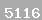# A hybrid computational approach to derive new ground-motion prediction equations

تاریخ ثبت : ۲۴-۱۰-۹۳    موضوع : مقالات منتشر شده در مجلات داراي رتبه علمي    امتیاز: 0 از 5  ( مجموع آرا : 0)
بازدید : ۱۳۴۹

A hybrid computational approach to derive new ground-motion prediction equations

SeyedMortezaTabatabaei

(The Institute of Higher Education of Eqbal Lahoori,Mashhad,Iran)

Engineering Applications of Artificial Intelligence, Elsevier, Volume 24, Issue 4, June 2011, Pages 717–732

رتبه مجله : ISI

Abstract

A novel hybrid method coupling genetic programming and orthogonal least squares, called GP/OLS, was employed to derive new ground-motion prediction equations (GMPEs). The principal ground-motion parameters formulated were peak ground acceleration (PGA), peak ground velocity (PGV) and peak ground displacement (PGD). The proposed GMPEs relate PGA, PGV and PGD to different seismic parameters including earthquake magnitude, earthquake source to site distance, average shear-wave velocity, and faulting mechanisms. The equations were established based on an extensive database of strong ground-motion recordings released by Pacific Earthquake Engineering Research Center (PEER). For more validity verification, the developed equations were employed to predict the ground-motion parameters of the Iranian plateau earthquakes. A sensitivity analysis was carried out to determine the contributions of the parameters affecting PGA, PGV and PGD. The sensitivity of the models to the variations of the influencing parameters was further evaluated through a parametric analysis. The obtained GMPEs are effectively capable of estimating the site ground-motion parameters. The equations provide a prediction performance better than or comparable with the attenuation relation-ships found in the literature. The derived GMPEs are remarkably simple and straightforward and can reliably be used for the pre-design purposes.

Keywords

Time-domain ground-motion parameters, Prediction equations, Genetic programming, Orthogonal least squares , Nonlinear modeling

کلمات کلیدی : مقاله ، isi ، سيد مرتضي طباطباييTime-domain ground-motion parameters, Prediction equations, Genetic programming, Orthogonal least squares , Nonlinear modeling ، پژوهشي ، اقبال لاهوري ، پرتال پژوهشي

ثبت نظر